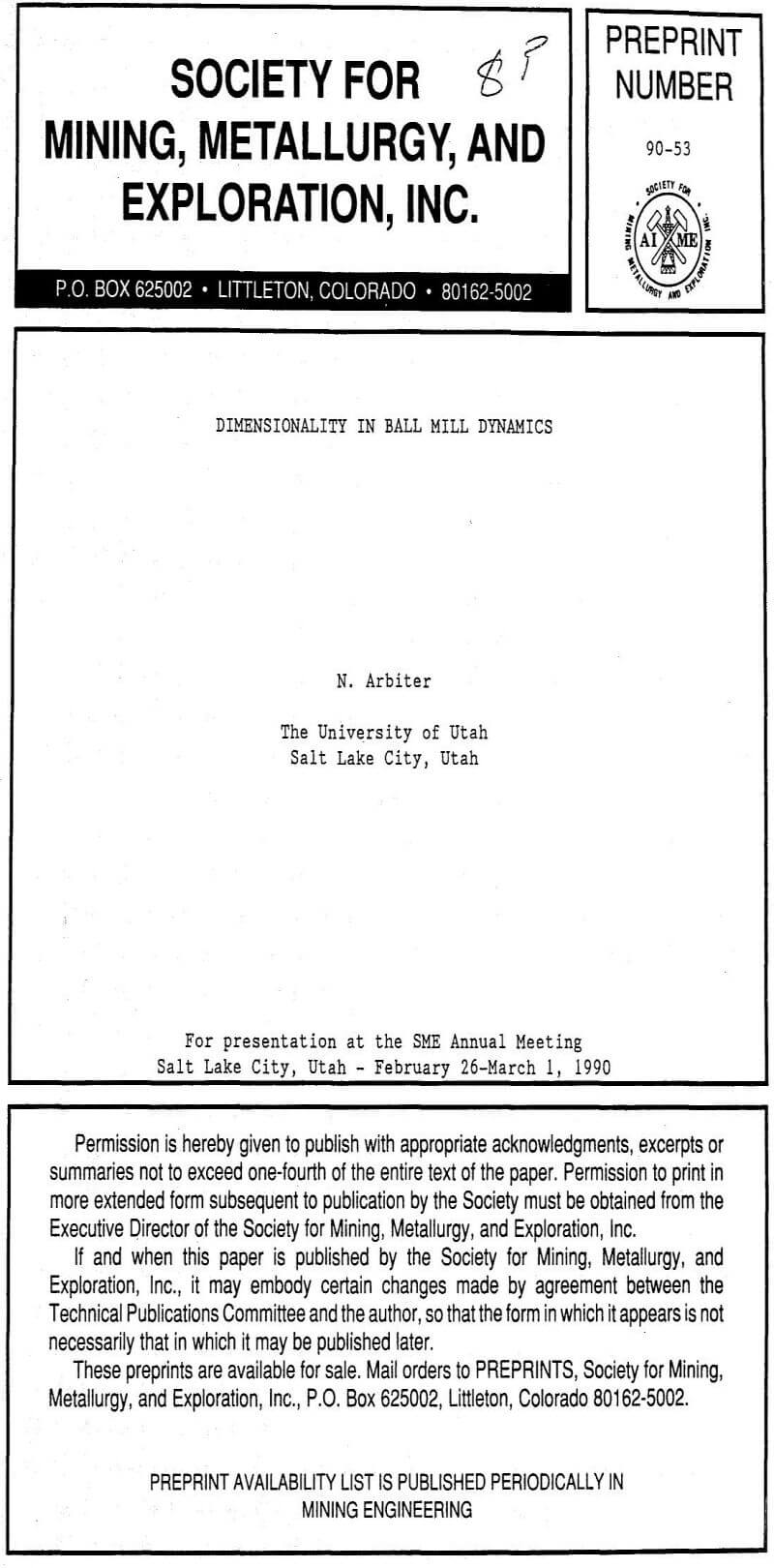# How to Reduce Ball Mill Overload Risk

Two different circumstances must be addressed for the reduction or elimination of overload risk: a) in existing operations, or b) in the design of new grinding circuits. The methods necessary are in the first case an adjustment of operating conditions; and in the second an adjustment of mill shapes.

Existing Operations: from Equation (1) for mill power with a system of fixed dimensions, power input, and ore properties, Equation (8) follows:

Fc (1 – Lf) Lf = C1………………………………………………………………(8)

The axial velocity equation at overload (Table 3) can be changed to:

Vax = C2/[0.50 – 0.66 Lf]………………………………………………………(9)With C2 determined by use of the Lf value at overload. As shown in Table 4, it is then possible to assume values for Vax/Vax to represent degrees of axial velocity reduction, along with lower values for Lf, and calculate the values of Fc necessary to hold power constant. The mill operating data in Table 2 show that at the already low Lf value for Lornex, little if any practical overload risk reduction is likely by this means. On the other hand, Sierrita’s Lf value of 0.48 suggests that major reductions in risk are possible without increasing the Fc value beyond the conventional operating range. Table 4 illustrates the procedures and results for three of the five mills in Table 2. For both Pinto Valley and B.C.L., useful reductions in overload risk are indicated by lowering the Lf values toward 0.30, while increasing Fc values toward 0.80. For Sierrita, risk reduction to 70 percent of the critical value results from lowering Lf to 0.36, while increasing Fc to 0.78 from 0.72. Pinto Valley, on the other hand, with an initial Lf value of 0.34, could obtain a risk reduction to only 88 percent of the critical value by lowering Lf to 0.28 and increasing Fc to 0.81—probably the maximum practical changes.

Design of New Mills: for determining the effects of dimensional changes on overload, a useful equation is obtained from the expression for axial velocity by substituting a value for the feed rate obtained from the power equation, with the assumption of constant Pt, Fc, Lf, and load and pulp density terms. This leads to:

vax = C1 D1.5L…………………………………………………………(10)

For a fixed average feed rate and Pt, Equation 1 becomes:

D2,5 L = C2……………………………………………………………..(11)

These two relationships permit calculating the changes necessary in D and L in order to reduce Vax by varying percentages of its critical value: Vax = 0.0769 m/s (15.1 ft/min).

Table 5 illustrates results, starting with 5.5 x 6.4 m and 5.5 x 8.5 m (18 x 21 ft; 18 x 28 ft) mills and reducing velocities from the overload value progressively to 60 percent of that value. It is notable that the largest diameter required is 6.8 m (22.5 ft), – much less than those current in North America for larger SAG or autogenous mills. This suggests that the construction of such mill sizes should not be a problem. While the specific power requirements would be higher than those necessary for SAG or autogenous mills due to higher load densities, there have been no difficulties in supplying the same power levels to the 5.5 m diameter mills in current use with L/D ratios up to 1.6. The  same argument holds for bearing loads, which would differ only marginally from those for current shapes at the same Lf values.

## Overload Risk and Mill Size/Capacity Effects

Table 6 illustrates the conditions required to obtain overload with smaller mills and lower mill throughputs under two assumed conditions:

1. Capacities and mill sizes are decreased progressively, with all operating conditions including circulating loads remaining constant, and L/D ratios increased to maintain the axial velocity at the critical value for overload.
2. Capacities and mill sizes are decreased; but L/D ratios are held constant, with circulating loads incr